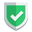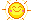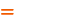本博客不欢迎：各种镜像采集行为，请尊重法律法规。 苏南大叔是谁？点此了解一个特立独行的世界

## 测试代码

``````import pandas as pd
from pandas import Series, DataFrame
df = DataFrame([
('虎子', 5, "dog"),
('老许', 3, "cat"),
],
columns=('name', 'age', 'class')
)
df.to_csv("test.csv")
df.to_csv("test2.csv", index=False)
df.to_csv("test3.csv", index=True)

``df.to_csv("test.csv")``

``df.to_csv("test2.csv", index=False)``

## 索引列参数index，默认值True

``````df.to_csv("test.csv")
df.to_csv("test.csv", index=True)``````

``df.to_csv("test.csv", index=False)``

``````df.to_csv("test.csv")

``df.to_csv("test.csv", header=False)``

## 参考文献

`python`自带的`csv`模块中，也有类似的功能`csvwriter`，具体可以参考下面的文章：

`pandas`官方的说明网页，见这里，注意链接中的版本号：

## 总结

`dataframe`数据转化为`csv`文件，可以使用遍历变量写入，也可以使用这个非常好用的`to_csv()`函数一次性写入。更多`pandas`文章，请点击苏南大叔的博客：如果本文对您有帮助，或者节约了您的时间，欢迎打赏瓶饮料，建立下友谊关系。本博客不欢迎：各种镜像采集行为。请尊重原创文章内容，转载请保留作者链接。【福利】 腾讯云最新爆款活动！1核2G云服务器首年50元！【服务】 一切外联事宜，授权管家处理。微信号：tudoumart【苹果】苹果生态程序员QQ群【787907940】，等您来加入【加群】加入QQ群【175454274】和大家一起讨论这个问题【源码】本文代码片段及相关软件，请点此获取更多信息【绝密】秘籍文章入口，仅传授于有缘之人pandas    csv